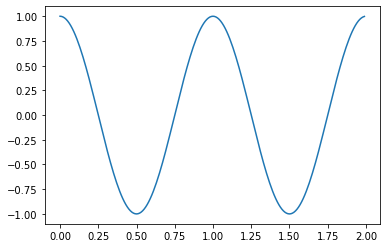👋 Hello! I'm Alphonsio the robot. Ask me a question, I'll try to answer.

# Simplest example with matplotlb

The following Python code is probably the simplest plot with Matplotlib:

import matplotlib.pyplot as plt
import numpy as np

# Prepare data y=cos(2π.x)
x = np.arange(0.0, 2.0, 0.01)
y = np.cos(2 * np.pi * x)

# Create figure and plot data
fig, ax = plt.subplots()
ax.plot(x, y)

The above code should display the following chart:Note that you can plot directly with ﻿plt.plot(x,y), but it's a good habit to collect ﻿fig and ﻿ax for future use (displaying grid, axis label, title ...).

More